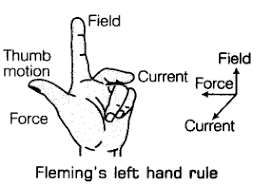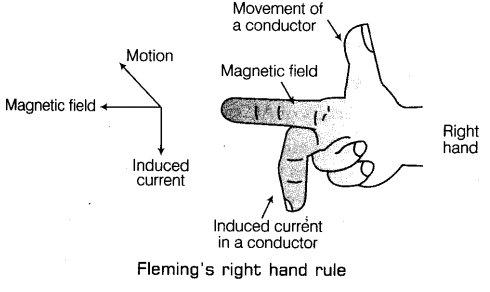3 Tutor System
Starting just at 265/hour

# State the rule to determine the direction of a (i) magnetic field produced around a straight conductor-carrying current, (ii) force experienced by a current-carrying straight conductor placed in a magnetic field which is perpendicular to it, and (iii) current induced in a coil due to its rotation in a magnetic field.

(i) Maxwell's right hand thumb rule Maxwell’s right hand rule states that if we are holding a current-carrying conductor in our right hand such that the thumb points in the direction of current, then the direction in which fingers encircle, gives the direction of magnetic lines. This rule is also called as Maxwell’s Corkscrew Rule.(ii) Fleming's left hand rule Fleming’s left hand rule states if the forefinger, thumb and middle finger of the left hand are stretched mutually perpendicular and the forefinger points in the direction of external magnetic field; middle finger indicates the direction of current, then the thumb points along the direction of force acting on the conductor.(iii) Fleming's right hand rule Fleming’s Right hand rule it states that the states that if we stretch the thumb, forefinger and the middle finger of right hand at right angles to one another in such a way that the forefinger points in the direction of magnetic field; then the thumb gives the direction of motion of conductor (force), forefinger indicates direction of magnetic field and the middle finger points the direction of induced current.